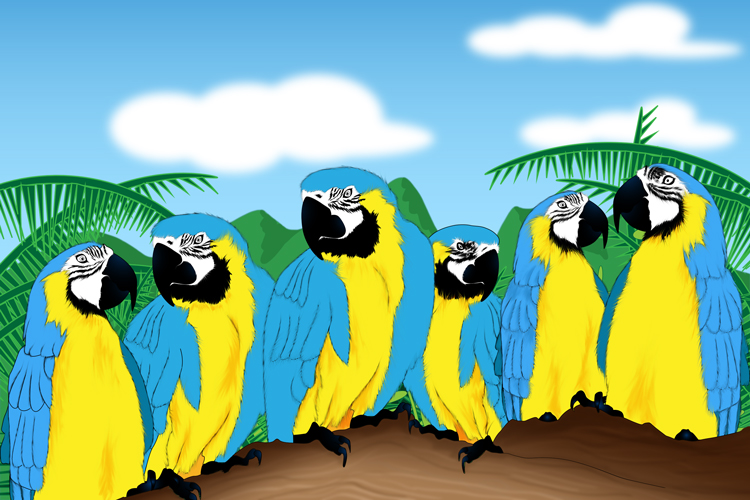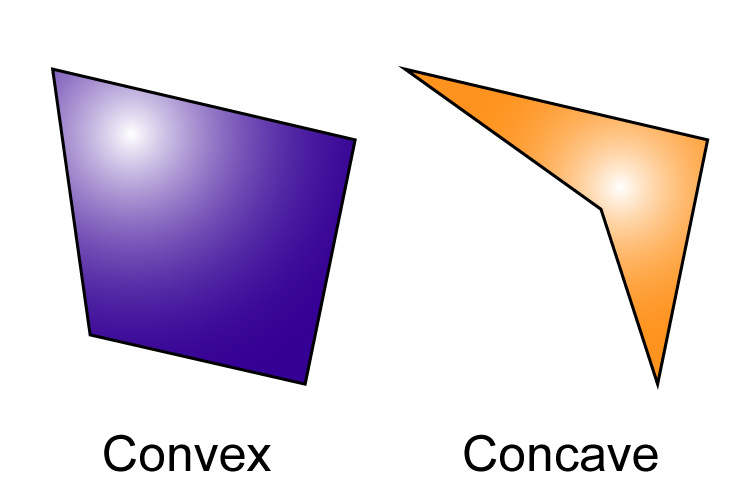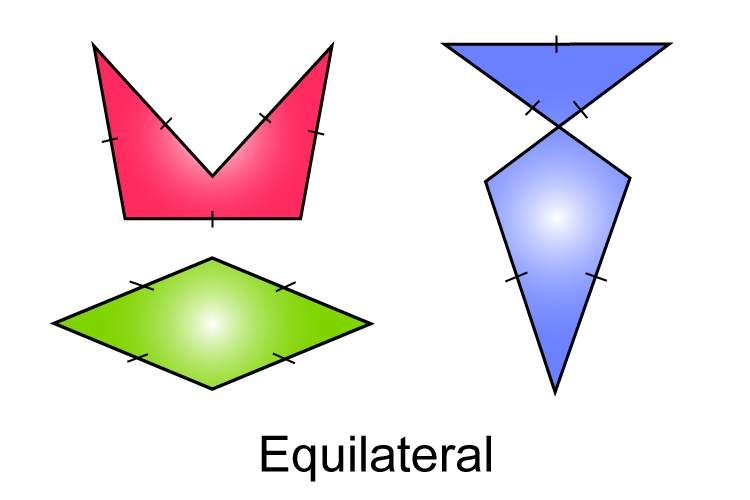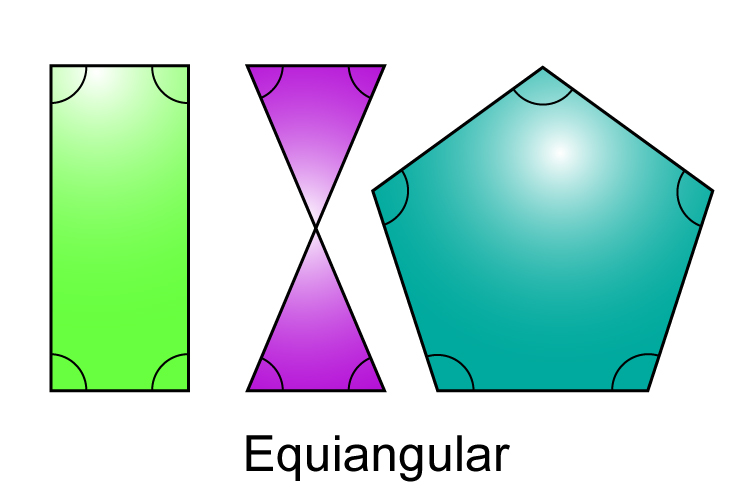# Polygon

Any shape made of straight edges/sides – many sides

## Poly = manyPolly the parrots were many and everywhere.

## Gon = sidesGone are the sideburns he’d had for years.

Example 1All the above are examples of polygons.

Example 2Polygons can be convex or concave.

Example 3Polygons where all the sides are equal length are known as equilateral polygons.

Example 4Polygons where all the internal angles are equal are known as equiangular polygons.